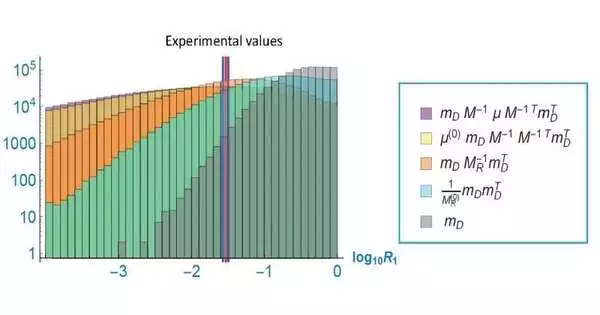Physics

# The riddle of neutrino mass is approached using random matrix theory.All that is left after breaking down any substance into smaller and smaller pieces to the point where it can no longer be divided any further is a particle. There are currently 12 different types of known elementary particles, which are composed of quarks and leptons, each of which has six distinct flavors. A variety of particles, such as the electron, muon, and tau neutrinos, are created from these flavors by dividing them into three generations, each of which contains one charged and one neutral lepton. In the Standard Model, a three-by-three matrix is used to represent the masses of the three generations of neutrinos.

Scientists from the Osaka Metropolitan University Graduate School of Science, under the direction of Professor Naoyuki Haba, examined the assortment of leptons that make up the neutrino mass matrix. As a result of the fact that neutrinos are known to have a smaller mass difference between generations than other elementary particles, the research team assumed that neutrinos have a mass difference between generations that is roughly equal. By allocating each component of the neutrino mass matrix at random, they examined it. The lepton flavor mixings are large; they were theoretically demonstrated using the random mass matrix model. Their research was released in the journal Progress in Theoretical and Experimental Physics.

“Understanding the properties of elementary particles leads to the exploration of the universe and, eventually, the grand theme of where we came from. There is a whole new realm of physics beyond the Standard Model’s unresolved puzzles.”

Professor Naoyuki Haba from the Osaka Metropolitan University Graduate School of Science,

Professor Haba said, “Clarifying the properties of elementary particles leads to the exploration of the universe and ultimately to the grand theme of where we came from. Beyond the remaining puzzles of the Standard Model, there is a completely new physics.”.

Studying the neutrino mass anarchy in the Dirac neutrino, seesaw, and double seesaw models led the researchers to the conclusion that the anarchy approach necessitates that the matrix’s measure adhere to the Gaussian distribution. The research team was able to demonstrate, as best they could at this point, why the calculation of the squared difference of the neutrino masses is closest to the experimental results in the case of the seesaw model with the random Dirac and Majorana matrices after considering several models of light neutrino masses where the matrix is composed of the product of several random matrices.

The neutrino mass hierarchy can be mathematically explained using random matrix theory, as we demonstrated in this study. Though mathematically incomplete, this proof is anticipated to be rigorously established as random matrix theory advances, according to Professor Haba. The essential nature of the three-generation copy structure of elementary particles, whose existence is still entirely unknown both theoretically and experimentally, will continue to be our challenge in the future.”.

More information: Naoyuki Haba et al, Neutrino mass square ratio and neutrinoless double-beta decay in random neutrino mass matrices, Progress of Theoretical and Experimental Physics (2023). DOI: 10.1093/ptep/ptad010

Topic : Article